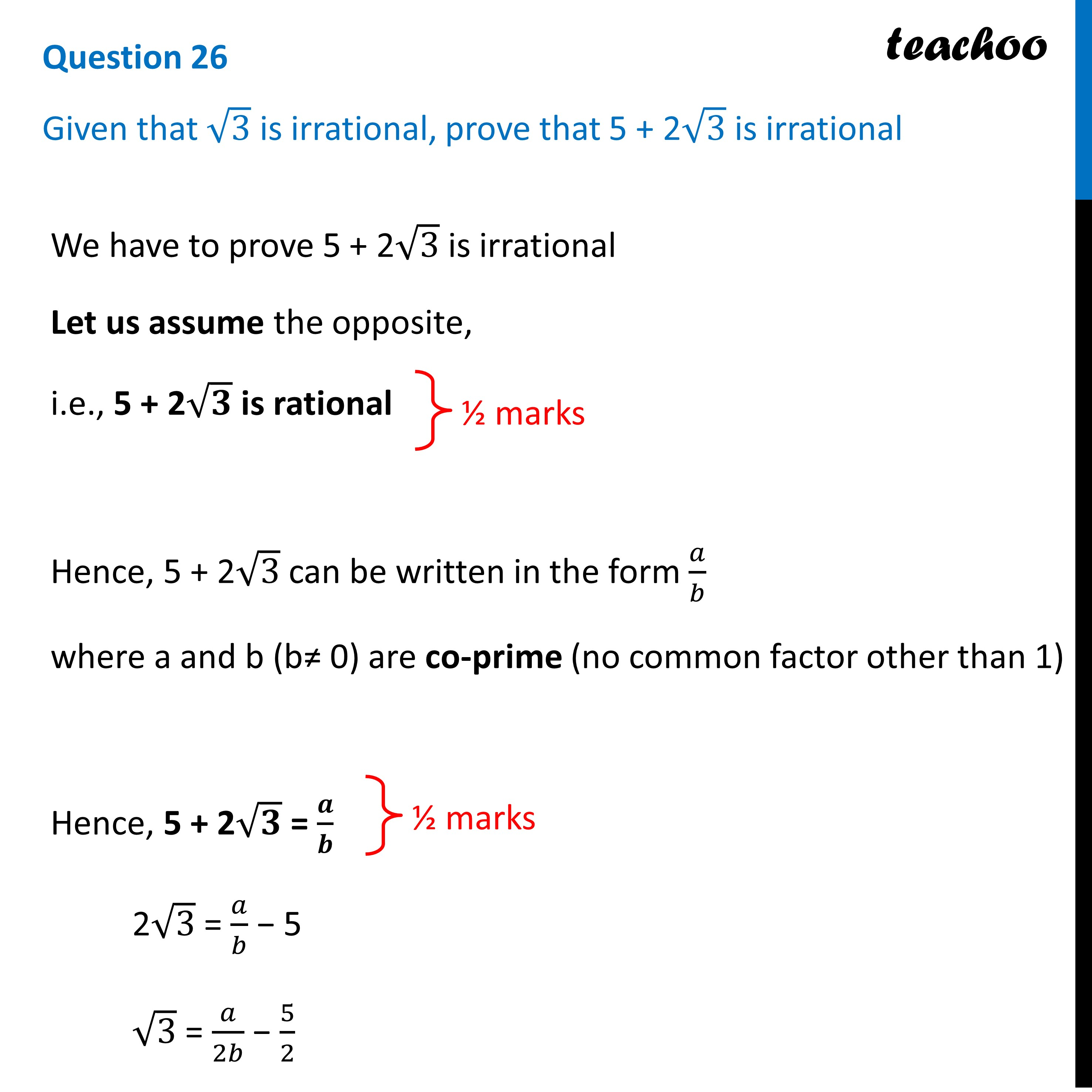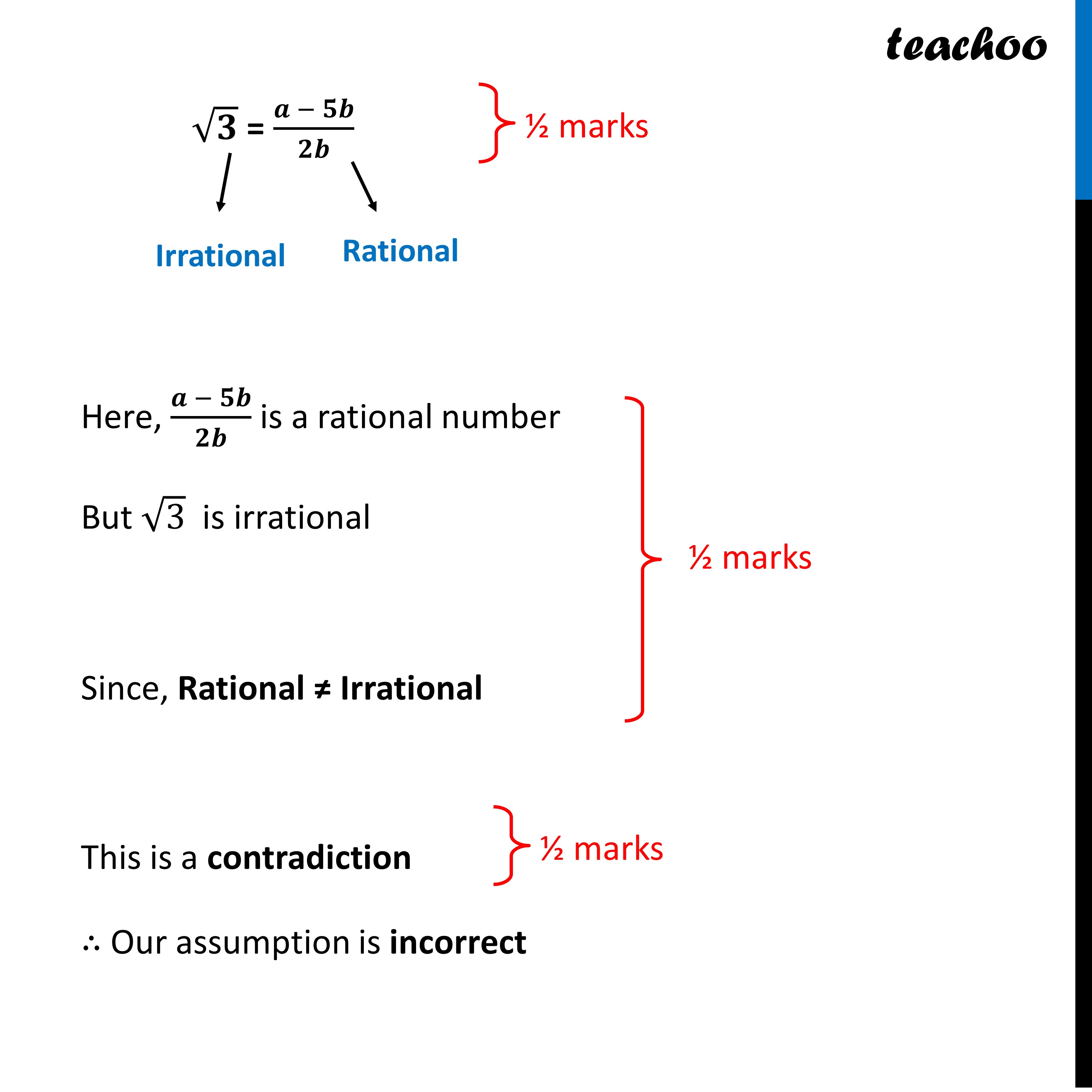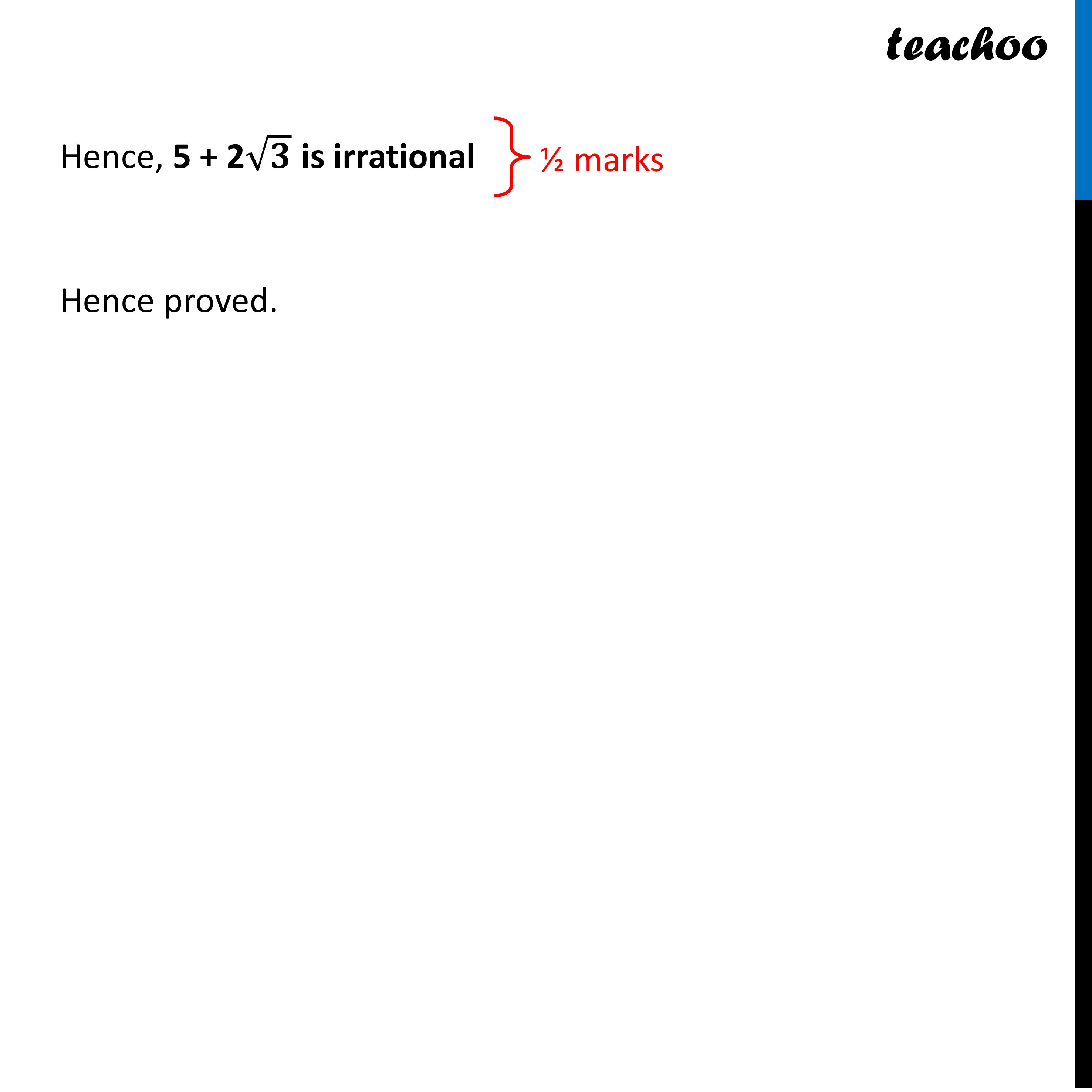CBSE Class 10 Sample Paper for 2023 Boards - Maths Standard

Class 10
Solutions of Sample Papers for Class 10 Boards

## Given that √3 is irrational, prove that 5 + 2√3 is irrational

This question is Similar to Question-27 CBSE Class 10 Sample Paper for 2021 Boards - Maths StandardLearn in your speed, with individual attention - Teachoo Maths 1-on-1 Class

### Transcript

Question 26 Given that √3 is irrational, prove that 5 + 2√3 is irrationalWe have to prove 5 + 2√3 is irrational Let us assume the opposite, i.e., 5 + 2√𝟑 is rational Hence, 5 + 2√3 can be written in the form 𝑎/𝑏 where a and b (b≠ 0) are co-prime (no common factor other than 1) Hence, 5 + 2√𝟑 = 𝒂/𝒃 2√3 = 𝑎/𝑏 − 5 √3 = 𝑎/2𝑏 − 5/2 √𝟑 = (𝒂 − 𝟓𝒃)/𝟐𝒃 Here, (𝒂 − 𝟓𝒃)/𝟐𝒃 is a rational number But √3 is irrational Since, Rational ≠ Irrational This is a contradiction ∴ Our assumption is incorrect Hence, 5 + 2√𝟑 is irrational Hence proved.